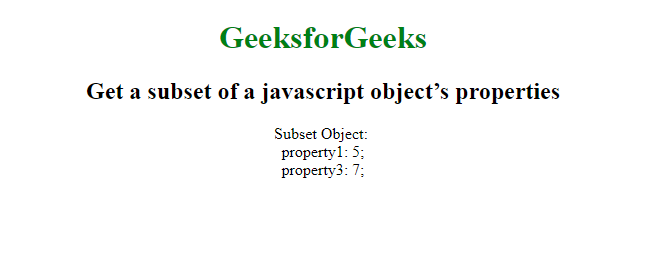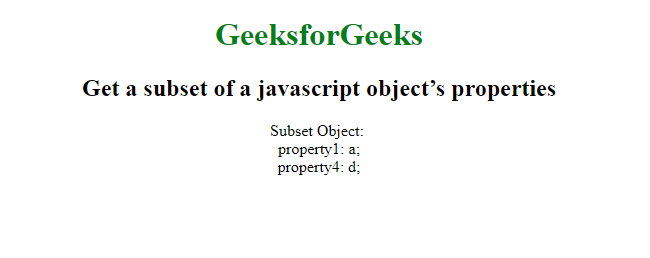# How to get a subset of a javascript object’s properties?

• Last Updated : 21 Nov, 2021

To get the subset of properties of a JavaScript Object, we make use of destructuring and Property Shorthand. The destructuring assignment syntax is a JavaScript expression that makes it possible to unpack values from arrays, or properties from objects, into distinct variables.
Syntax:

`subset = (({a, c}) => ({a, c}))(obj);`

Example1: Get subset of a javascript object’s properties using destructuring assignment.

Hey geek! The constant emerging technologies in the world of web development always keeps the excitement for this subject through the roof. But before you tackle the big projects, we suggest you start by learning the basics. Kickstart your web development journey by learning JS concepts with our JavaScript Course. Now at it's lowest price ever!

## html

 `` `<``html``>`   `<``head``>` `    ``<``title``>` `      ``Get a subset of a javascript object’s properties` `  ``` ``   `<``body``>` `    ``<``center``>` `        ``<``h1` `style``=``"color:green"``>` `          ``GeeksforGeeks` `      ``` `        ``<``h2``>` `          ``Get a subset of a javascript object’s properties` `      ``` `        ``<``script``>` `            ``obj = {` `                ``property1: 5,` `                ``property2: 6,` `                ``property3: 7` `            ``};` `            ``subset = (({` `                ``property1, property3` `            ``}) => ({` `                ``property1, property3` `            ``}))(obj);`   `            ``var output = 'Subset Object: <``br``>';` `            ``for (var property in subset) {` `                ``output += property + ': ' + subset[property] + ';<``br``>';` `            ``}` `            ``document.write(output);` `        ``` `    ``` ``   ``

Output:In ES6 there is a very concise way to do this using destructing. Destructing allows you to easily add on to objects and make subset objects.
Syntax:

```const {c, d, ...partialObject} = object;
const subset = {c, d};```

Example 2: Get subset of a javascript object’s properties using destructuring assignment.

## html

 `` `<``html``>`   `<``head``>` `    ``<``title``>` `      ``Get a subset of a javascript object’s properties` `  ``` ``   `<``body``>` `    ``<``center``>` `        ``<``h1` `style``=``"color:green"``>` `          ``GeeksforGeeks` `      ``` `        ``<``h2``>` `          ``Get a subset of a javascript object’s properties` `      ``` `        ``<``script``>` `            ``const object = {` `                ``property1: 'a',` `                ``property2: 'b',` `                ``property3: 'c',` `                ``property4: 'd',` `            ``}`   `            ``const {` `                ``property1,` `                ``property4,` `                ``...pObject` `            ``}`   `            ``= object;` `            ``const subset = {` `                ``property1,` `                ``property4` `            ``}`   `            ``;` `            ``var output = 'Subset Object: <``br``>';` `            ``for (var property in subset) {` `                ``output += property + ': ' +` `                    ``subset[property] + ';<``br``>';` `            ``}`   `            ``document.write(output);` `        ``` `    ``` ``   ``

Output:My Personal Notes arrow_drop_up
Recommended Articles
Page :